# Dispersion (water waves)

Dispersion (water waves)

In fluid dynamics, dispersion of water waves generally refers to frequency dispersion, which means that waves of different wavelengths travel at different phase speeds. Water waves, in this context, are waves propagating on the water surface, and forced by gravity and surface tension. As a result, water with a free surface is generally considered to be a dispersive medium.

Surface gravity waves, moving under the forcing by gravity, propagate faster for increasing wavelength. For a given wavelength, gravity waves in deeper water have a larger phase speed than in shallower water. In contrast with this, capillary waves only forced by surface tension, propagate faster for shorter wavelengths.

Besides frequency dispersion, water waves also exhibit amplitude dispersion. This is a nonlinear effect, by which waves of larger amplitude have a different phase speed from small-amplitude waves.

## Frequency dispersion for surface gravity waves

This section is about frequency dispersion for waves on a fluid layer forced by gravity, and according to linear theory.

### Wave propagation and dispersion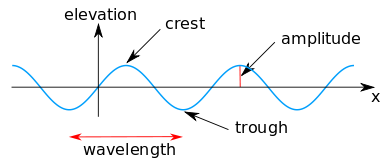Sinusoidal wave.

The simplest propagating wave of unchanging form is a sine wave. A sine wave with water surface elevation η( x, t ) is given by:$\eta(x,t) = a \sin \left( \theta(x,t) \right),\,$

where a is the amplitude (in metres) and θ = θ( x, t ) is the phase function (in radians), depending on the horizontal position ( x , in metres) and time ( t , in seconds):$\theta = 2\pi \left( \frac{x}{\lambda} - \frac{t}{T} \right) = k x - \omega t,$   with$k = \frac{2\pi}{\lambda}$   and$\omega = \frac{2\pi}{T},$

where:

Characteristic phases of a water wave are:

• the upward zero-crossing at θ = 0,
• the wave crest at θ = ½ π,
• the downward zero-crossing at θ = π and
• the wave trough at θ = 1½ π.

A certain phase repeats itself after an integer m multiple of : sin(θ) = sin(θ+m•2π).

Essential for water waves, and other wave phenomena in physics, is that free propagating waves of non-zero amplitude only exist when the angular frequency ω and wavenumber k (or equivalently the wavelength λ and period T ) satisfy a functional relationship: the frequency dispersion relation$\omega^2 = \Omega^2(k).\,$

The dispersion relation has two solutions: ω = +Ω(k) and ω = -Ω(k), corresponding to waves travelling in the positive or negative x–direction. The dispersion relation will in general depend on several other parameters in addition to the wavenumber k. For gravity waves, according to linear theory, these are the acceleration by gravity and the water depth.

An initial wave phase θ = θ0 propagates as a function of space and time. Its subsequent position is given by:$x = \frac{\lambda}{T}\, t + \frac{\lambda}{2\pi}\, \theta_0 = \frac{\omega}{k}\, t + \frac{\theta_0}{k}.$

This shows that the phase moves with the velocity:$c_p = \frac{\lambda}{T} = \frac{\omega}{k} = \frac{\Omega(k)}{k},$

which is called the phase velocity.

### Phase velocity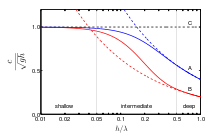Dispersion of gravity waves on a fluid surface. Phase and group velocity divided by shallow-water phase velocity √(gh) as a function of relative depth h / λ. Blue lines (A): phase velocity; Red lines (B): group velocity; Black dashed line (C): phase and group velocity √(gh) valid in shallow water. Drawn lines: dispersion relation valid in arbitrary depth. Dashed lines (blue and red): deep water limits.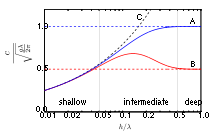Dispersion of gravity waves on a fluid surface. Phase and group velocity divided by deep-water phase velocity √(½ gλ / π) as a function of relative depth h / λ. Blue lines (A): phase velocity; Red lines (B): group velocity; Black dashed line (C): phase and group velocity √(gh) valid in shallow water. Drawn lines: dispersion relation valid in arbitrary depth. Dashed lines (blue and red): deep water limits.

A sinusoidal wave, of small surface-elevation amplitude and with a constant wavelength, propagates with the phase velocity, also called celerity or phase speed. While the phase velocity is a vector and has an associated direction, celerity or phase speed refer only to the magnitude of the phase velocity. According to linear theory for waves forced by gravity, the phase speed depends on the wavelength and the water depth. For a fixed water depth, long waves (with large wavelength) propagate faster than shorter waves.

In the left figure, it can be seen that shallow water waves, with wavelengths λ much larger than the water depth h, travel with the phase velocity$c_p = \sqrt{gh} \qquad \text{(shallow water),}\,$

with g the acceleration by gravity and cp the phase speed. Since this shallow-water phase speed is independent of the wavelength, shallow water waves do not have frequency dispersion.

Using another normalization for the same frequency dispersion relation, the figure on the right shows that in deep water, with water depth h larger than half the wavelength λ (so for h/λ > 0.5), the phase velocity cp is independent of the water depth:$c_p = \sqrt{\frac{g\lambda}{2\pi}} = \frac{g}{2\pi} T \qquad \text{(deep water),}$

with T the wave period (the reciprocal of the frequency f, T=1/f ). So in deep water the phase speed increases with the wavelength, and with the period.

Since the phase speed satisfies cp = λ/T = λf, wavelength and period (or frequency) are related. For instance in deep water:$\lambda = \frac{g}{2\pi}T^2 \qquad \text{(deep water).}$

The dispersion characteristics for intermediate depth are given below.

### Group velocity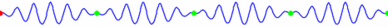Frequency dispersion in bichromatic groups of gravity waves on the surface of deep water. The red dot moves with the phase velocity, and the green dots propagate with the group velocity.

Interference of two sinusoidal waves with slightly different wavelengths, but the same amplitude and propagation direction, results in a beat pattern, called a wave group. As can be seen in the animation, the group moves with a group velocity cg different from the phase velocity cp, due to frequency dispersion.

The group velocity is depicted by the red lines (marked B) in the two figures above. In shallow water, the group velocity is equal to the shallow-water phase velocity. This is because shallow water waves are not dispersive. In deep water, the group velocity is equal to half the phase velocity: cg = ½ cp.

The group velocity also turns out to be the energy transport velocity. This is the velocity with which the mean wave energy is transported horizontally in a narrow-band wave field.

In case of a the group velocity different from the phase velocity, a consequence is that the number of waves counted in a wave group is different when counted from a snapshot in space at a certain moment, from when counted in time from the measured surface elevation at a fixed position. Consider a wave group of length Λg and group duration of τg. The group velocity is:$c_g = \frac{\Lambda_g}{\tau_g}.$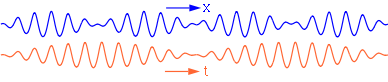The number of waves per group as observed in space at a certain moment (upper blue line), is different from the number of waves per group seen in time at a fixed position (lower orange line), due to frequency dispersion.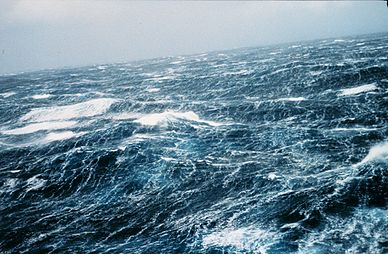North Pacific storm waves as seen from the NOAA M/V Noble Star, Winter 1989.

The number of waves in a wave group, measured in space at a certain moment is: Λg / λ. While measured at a fixed location in time, the number of waves in a group is: τg / T. So the ratio of the number of waves measured in space to those measured in time is:$\tfrac{\text{No. of waves in space}}{\text{No. of waves in time}} = \frac{\Lambda_g / \lambda}{\tau_g / T} = \frac{\Lambda_g}{\tau_g} \cdot \frac{T}{\lambda} = \frac{c_g}{c_p}.$

So in deep water, with cg = ½ cp, a wave group has twice as many waves in time as it has in space.

The water surface elevation η(x,t), as a function of horizontal position x and time t, for a bichromatic wave group of full modulation can be mathematically formulated as:$\eta = a\, \sin \left( k_1 x - \omega_1 t \right) + a\, \sin \left( k_2 x - \omega_2 t \right),$

with:

• a the wave amplitude of each frequency component in metres,
• k1 and k2 the wave number of each wave component, in radians per metre, and
• ω1 and ω2 the angular frequency of each wave component, in radians per second.

Both ω1 and k1, as well as ω2 and k2, have to satisfy the dispersion relation:$\omega_1^2 = \Omega^2(k_1)\,$   and$\omega_2^2 = \Omega^2(k_2).\,$

Using trigonometric identities, the surface elevation is written as:$\eta= \left[ 2\, a\, \cos \left( \frac{k_1 - k_2}{2} x - \frac{\omega_1 - \omega_2}{2} t \right) \right]\; \cdot\; \sin \left( \frac{k_1 + k_2}{2} x - \frac{\omega_1 + \omega_2}{2} t \right).$

The part between square brackets is the slowly-varying amplitude of the group, with group wave number ½ ( k1 - k2 ) and group angular frequency ½ ( ω1 - ω2 ). As a result, the group velocity is, for the limit k1 → k2 :$c_g = \lim_{k_1\, \to\, k_2} \frac{\omega_1 - \omega_2}{k_1 - k_2} = \lim_{k_1\, \to\, k_2} \frac{\Omega(k_1) - \Omega(k_2)}{k_1 - k_2} = \frac{\text{d}\Omega(k)}{\text{d}k}.$

Wave groups can only be discerned in case of a narrow-banded signal, with the wave-number difference k1 - k2 small compared to the mean wave number ½ (k1 + k2).

### Multi-component wave patternsFrequency dispersion of surface gravity waves on deep water. The superposition (dark blue line) of three sinusoidal wave components (light blue lines) is shown.

The effect of frequency dispersion is that the waves travel as a function of wavelength, so that spatial and temporal phase properties of the propagating wave are constantly changing. For example, under the action of gravity, water waves with a longer wavelength travel faster than those with a shorter wavelength.

While two superimposed sinusoidal waves, called a bichromatic wave, have an envelope which travels unchanged, three or more sinusoidal wave components result in a changing pattern of the waves and their envelope. A sea state – that is: real waves on the sea or ocean – can be described as a superposition of many sinusoidal waves with different wavelengths, amplitudes, initial phases and propagation directions. Each of these components travels with its own phase velocity, in accordance with the dispersion relation. The statistics of such a surface can be described by its power spectrum.

### Dispersion relation

In the table below, the dispersion relation ω2 = [Ω(k)]2 between angular frequency ω = 2π / T and wave number k = 2π / λ is given, as well as the phase and group speeds.

Deep water corresponds with water depths larger than half the wavelength, which is the common situation in the ocean. In deep water, longer period waves propagate faster and transport their energy faster. The deep-water group velocity is half the phase velocity. In shallow water, for wavelengths larger than twenty times the water depth, as found quite often near the coast, the group velocity is equal to the phase velocity.

## History

The full linear dispersion relation was first found by Pierre-Simon Laplace, although there were some errors in his solution for the linear wave problem. The complete theory for linear water waves, including dispersion, was derived by George Biddell Airy and published in about 1840. A similar equation was also found by Philip Kelland at around the same time (but making some mistakes in his derivation of the wave theory).

The shallow water (with small h / λ) limit, ω2 = gh k2, was derived by Joseph Louis Lagrange.

## Surface tension effects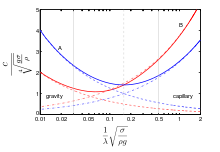Dispersion of gravity-capillary waves on the surface of deep water. Phase and group velocity divided by$\sqrt{g\sigma/\rho}$ as a function of relative wavelength$\frac{1}{\lambda}\sqrt{\sigma/(\rho g)}$.
Blue lines (A): phase velocity, Red lines (B): group velocity.
Drawn lines: dispersion relation for gravity-capillary waves.
Dashed lines: dispersion relation for deep-water gravity waves.
Dash-dot lines: dispersion relation valid for deep-water capillary waves.

In case of gravity-capillary waves, where surface tension affects the waves, the dispersion relation becomes:$\omega^2 = \left( g k + \frac{\sigma}{\rho} k^3 \right) \tanh (kh),$

with σ the surface tension (in N/m).

## Nonlinear effects

### Shallow water

Amplitude dispersion effects appear for instance in the solitary wave (or soliton): a single hump of water traveling with constant velocity in shallow water with a horizontal bed. The single soliton solution of the Korteweg–de Vries equation, of wave height H in water depth h far away from the wave crest, travels with the velocity:$c_p = c_g = \sqrt{g(h+H)}.$

So for this nonlinear gravity wave it is the total water depth under the wave crest that determines the speed, with higher waves traveling faster than lower waves. Note that soliton solutions only exist for positive values of H, solitary gravity waves of depression do not exist.

### Deep water

The linear dispersion relation – unaffected by wave amplitude – is for nonlinear waves also correct at the second order of the perturbation theory expansion, with the orders in terms of the wave steepness k A (where A is wave amplitude). To the third order, and for deep water, the dispersion relation is$\omega^2 = gk \left[1+(kA)^2\right].$

This implies that large waves travel faster than small ones of the same frequency. This is only noticeable when the wave steepness k A is large.

## Waves on a mean current: Doppler shift

Water waves on a mean flow (so a wave in a moving medium) experience a Doppler shift. Suppose the dispersion relation for a non-moving medium is:$\omega^2 = \Omega^2(k),\,$

with k the wavenumber. Then for a medium with mean velocity vector V, the dispersion relationship with Doppler shift becomes:$\left( \omega - \bold k \cdot \bold V \right)^2 = \Omega^2(k),$

where k is the wavenumber vector, related to k as: k = |k|. The inner product kV is equal to: kV = kV cos α, with V the length of the mean velocity vector V: V = |V|. And α the angle between the wave propagation direction and the mean flow direction. For waves and current in the same direction, kV=kV.

### Dispersive water-wave models

Wikimedia Foundation. 2010.

### Look at other dictionaries:

• Boussinesq approximation (water waves) — In fluid dynamics, the Boussinesq approximation for water waves is an approximation valid for weakly non linear and fairly long waves. The approximation is named after Joseph Boussinesq, who first derived them in response to the observation by… …   Wikipedia

• Dispersion — may refer to: In physics: The dependence of wave velocity on frequency or wavelength: Dispersion (optics), for light waves Dispersion (water waves) Acoustic dispersion, for sound waves Dispersion relation, the mathematical description of… …   Wikipedia

• Dispersion relation — The refraction of a light in a prism is due to dispersion. In physics and electrical engineering, dispersion most often refers to frequency dependent effects in wave propagation. Note, however, that there are several other uses of the word… …   Wikipedia

• dispersion — /di sperr zheuhn, sheuhn/, n. 1. Also, dispersal. an act, state, or instance of dispersing or of being dispersed. 2. Optics. a. the variation of the index of refraction of a transparent substance, as glass, with the wavelength of light, with the… …   Universalium

• Color of water — For the book by James McBride, see The Color of Water. Water portal …   Wikipedia

• Deep ocean water — (DOW) is the name for cold, salty water found deep below the surface of Earth s oceans. Ocean water differs in temperature and salinity, with warm, relatively non salty water found at the surface, and very cold salty water found deeper below the… …   Wikipedia

• Capillary wave — A capillary wave is a wave travelling along the interface between two fluids, whose dynamics are dominated by the effects of surface tension.Capillary waves are common in nature and home, and are often referred to as ripple. The wavelength of… …   Wikipedia

• List of wave topics — This is a list of wave topics.0 ndash;9*21 cm lineA*Abbe prism *absorption spectrum *acoustics *Airy disc *Airy wave theory *Alfvén wave *Alpha waves *amphidromic point *amplitude *amplitude modulation *analog sound vs. digital sound *animal… …   Wikipedia

• Korteweg–de Vries equation — In mathematics, the Korteweg–de Vries equation (KdV equation for short) is a mathematical model of waves on shallow water surfaces. It is particularly notable as the prototypical example of an exactly solvable model, that is, a non linear partial …   Wikipedia

• Dispersive partial differential equation — In mathematics, a dispersive partial differential equation or dispersive PDE is a partial differential equation that is dispersive. In this context, dispersion means that waves of different wavelength propagate at different phase velocities.… …   Wikipedia